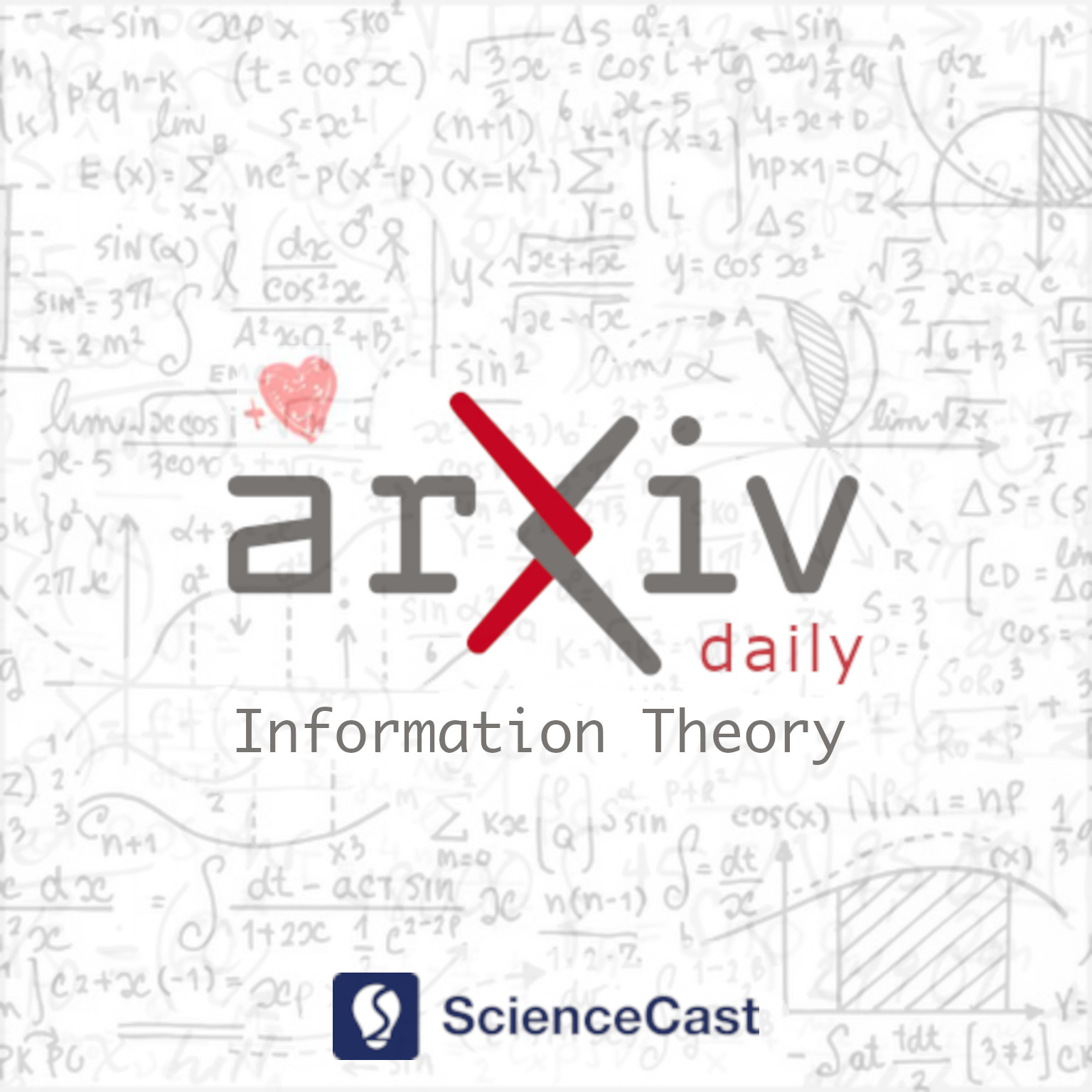##### 1.Entropy-Based Energy Dissipation Analysis of Mobile Communication Systems

Authors:Litao Yan, Xiaohu Ge

Abstract: Compared with the energy efficiency of conventional mobile communication systems, the energy efficiency of fifth generation (5G) communication systems has been improved more than 30 times. However, the energy consumption of 5G communication systems is 3 times of the energy consumption of fourth generation (4G) communication systems when the wireless traffic is increased more than 100 times in the last decade. It is anticipated that the traffic of future sixth generation (6G) communication systems will keep an exponential growth in the next decade. It is a key issue how much space is left for improving of energy efficiency in mobile communication systems. To answer the question, an entropy-based energy dissipation model based on nonequilibrium thermodynamics is first proposed for mobile communication systems. Moreover, the theoretical minimal energy dissipation limits are derived for typical modulations in mobile communication systems. Simulation results show that the practical energy dissipation of information processing and information transmission is three and seven orders of magnitude away from the theoretical minimal energy dissipation limits in mobile communication systems, respectively. These results provide some guidelines for energy efficiency optimization in future mobile communication systems.

##### 2.Faster List Decoding of AG Codes

Authors:Peter Beelen, Vincent Neiger

Abstract: In this article, we present a fast algorithm performing an instance of the Guruswami-Sudan list decoder for algebraic geometry codes. We show that any such code can be decoded in $\tilde{O}(s^2\ell^{\omega-1}\mu^{\omega-1}(n+g) + \ell^\omega \mu^\omega)$ operations in the underlying finite field, where $n$ is the code length, $g$ is the genus of the function field used to construct the code, $s$ is the multiplicity parameter, $\ell$ is the designed list size and $\mu$ is the smallest positive element in the Weierstrass semigroup of some chosen place.

##### 3.Resource Allocation and Passive Beamforming for IRS-assisted URLLC Systems

Authors:Yangyi Zhang, Xinrong Guan, Zhi Ji, Qingqing Wu, Yueming Cai

Abstract: In this correspondence, we investigate an intelligent reflective surface (IRS) assisted downlink ultra-reliable and low-latency communication (URLLC) system, where an access point (AP) sends short packets to multiple devices with the help of an IRS. Specifically, a performance comparison between the frequency division multiple access (FDMA) and time division multiple access (TDMA) is conducted for the considered system, from the perspective of average age of information (AoI). Aiming to minimize the maximum average AoI among all devices by jointly optimizing the resource allocation and passive beamforming. However, the formulated problem is difficult to solve due to the non-convex objective function and coupled variables. Thus, we propose an alternating optimization based algorithm by dividing the original problem into two sub-problems which can be efficiently solved. Simulation results show that TDMA can achieve lower AoI by exploiting the time-selective passive beamforming of IRS for maximizing the signal to noise ratio (SNR) of each device consecutively. Moreover, it also shows that as the length of information bits becomes sufficiently large as compared to the available bandwidth, the proposed FDMA transmission scheme becomes more favorable instead, due to the more effective utilization of bandwidth.